﻿ 基于PSO-Fuzzy-PID的四旋翼飞行器悬停控制

基于PSO-Fuzzy-PID的四旋翼飞行器悬停控制Hover Control for the Quadrotor Aircraft Based on PSO-Fuzzy-PID

Abstract: In order to suit the changes of quadrotor aircraft control system and the outside environment, a kind of hover control method for the quadrotor aircraft based on PSO-Fuzzy-PID is proposed in this paper. The model of the quadrotor aircraft is built, and the PSO-Fuzzy-PID control method, composed of particle swarm optimization algorithm and fuzzy adaptive PID control method, is in-troduced to control the hover of the quadrotor aircraft, adjusting the three parameters of fuzzy adaptive PID online by particle swarm optimization algorithm. The MATLAB simulation results show that the above method is superior to classical PID control method and fuzzy adaptive PID control method both on performance and adaptability.

1. 引言

2. 四旋翼飞行器建模

$R=\left[\begin{array}{ccc}C\psi & -S\psi & 0\\ S\psi & C\psi & 0\\ 0& 0& 1\end{array}\right]\ast \left[\begin{array}{ccc}C\theta & 0& S\theta \\ 0& 1& 0\\ -S\theta & 0& C\theta \end{array}\right]\ast \left[\begin{array}{ccc}1& 0& 0\\ 0& C\varphi & -S\varphi \\ 0& S\varphi & C\varphi \end{array}\right]=\left[\begin{array}{ccc}C\psi C\theta & C\psi S\theta S\varphi -S\psi C\varphi & S\varphi S\psi +C\psi S\theta C\varphi \\ C\theta S\psi & C\varphi C\psi +S\varphi S\theta S\psi & S\psi S\theta C\varphi -C\psi S\varphi \\ -S\theta & S\varphi C\theta & C\varphi C\theta \end{array}\right]$ (1)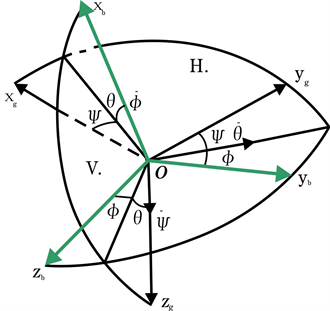Figure 1. Mathematical description of quadrotor system

$\left\{\begin{array}{l}{F}_{B}={\left[{F}_{x},{F}_{y},{F}_{z}\right]}^{\text{T}}={\left[0,0,{U}_{1}\right]}^{\text{T}}\\ {U}_{1}={F}_{1}+{F}_{2}+{F}_{3}+{F}_{4}\end{array}$ (2)

${F}_{E}={\left[{F}_{X},{F}_{Y},{F}_{Z}\right]}^{\text{T}}={U}_{1}{\left[S\varphi S\psi +C\psi S\theta C\varphi ,S\psi S\theta C\varphi -C\psi S\varphi ,C\varphi C\theta \right]}^{\text{T}}$ (3)

$\left\{\begin{array}{l}\stackrel{¨}{X}={U}_{1}\left(S\varphi S\psi +C\psi S\theta C\varphi \right)/m\\ \stackrel{¨}{Y}={U}_{1}\left(S\psi S\theta C\varphi -C\psi S\varphi \right)/m\\ \stackrel{¨}{Z}=\frac{{U}_{1}C\varphi C\theta }{m}-g\end{array}$ (4)

${I}_{X},{I}_{Y},{I}_{Z}$ 为四旋翼三个轴上的转动惯量， ${U}_{2},{U}_{3},{U}_{4}$ 为四旋翼的滚转力矩、俯仰力矩和偏航力矩，假设四旋翼的结构是完全对称的，忽略陀螺效应和空气阻力，四旋翼的小角度运动模型如下：

$\left\{\begin{array}{l}\stackrel{¨}{\varphi }=\frac{{U}_{2}}{{I}_{X}},\left({U}_{2}=kb\left({w}_{2}^{2}-{w}_{4}^{2}\right)\right)\\ \stackrel{¨}{\theta }=\frac{{U}_{3}}{{I}_{Y}},\left({U}_{3}=kb\left({w}_{3}^{2}-{w}_{1}^{2}\right)\right)\\ \stackrel{¨}{\phi }=\frac{{U}_{4}}{{I}_{Z}},\left({U}_{2}=kb\left({w}_{1}^{2}+{w}_{3}^{2}-{w}_{4}^{2}-{w}_{2}^{2}\right)\right)\end{array}$ (5)

$\left\{\begin{array}{l}\stackrel{¨}{X}={U}_{1}\left(S\varphi S\psi +C\psi S\theta C\varphi \right)/m\\ \stackrel{¨}{Y}={U}_{1}\left(S\psi S\theta C\varphi -C\psi S\varphi \right)/m\\ \stackrel{¨}{Z}=\left({U}_{1}C\varphi C\theta /m\right)-g\\ \stackrel{¨}{\varphi }={U}_{2}/{I}_{X}\\ \stackrel{¨}{\theta }={U}_{3}/{I}_{Y}\\ \stackrel{¨}{\phi }={U}_{4}/{I}_{Z}\end{array}$ (6)

3. 模糊自适应PID控制系统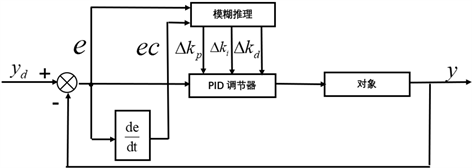Figure 2. The diagram of the fuzzy adaptive PID control structure

${k}_{p}={k}_{p0}+\Delta {k}_{p},{k}_{i}={k}_{i0}+\Delta {k}_{i},{k}_{d}={k}_{d0}+\Delta {k}_{d}$ (7)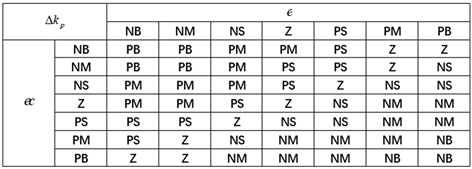Figure 3. ${k}_{p}$ setting rules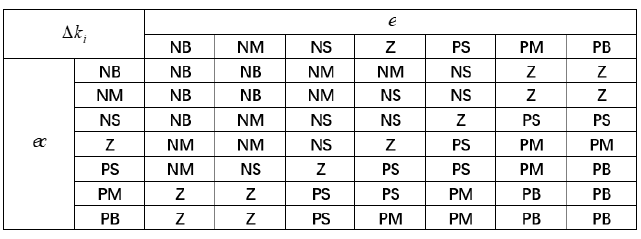Figure 4. ${k}_{i}$ setting rules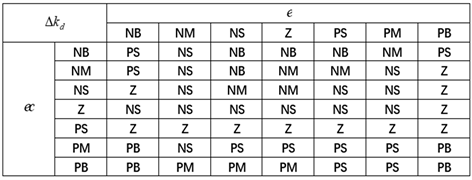Figure 5. ${k}_{d}$ setting rules

4. 基于粒子群优化的模糊自适应PID参数优化结构

${v}_{k+1}=\omega {v}_{k}+{c}_{1}rand\left(\text{ }\right)\left(pbes{t}_{k}-{x}_{k}\right)+{c}_{2}rand\left(\text{ }\right)\left(gbes{t}_{k}-{x}_{k}\right)$ (8)

${x}_{k+1}={x}_{k}+{v}_{k+1}$ (9)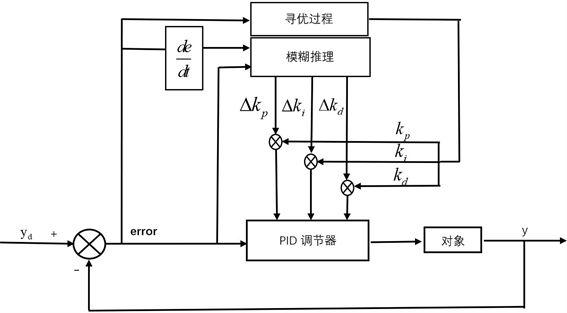Figure 6. Fuzzy PID control block diagram based on PSOFigure 7. Flow chart of fuzzy PID simulation based on PSO

1) 粒子群优化算法初始化。待优化的参数分别为比例 ${k}_{p}$ 、积分 ${k}_{i}$ 、微分 ${k}_{d}$，维数为3；设定粒子群算法的惯性权重 $\omega$ 为1；为了方便优化，本文构造50个粒子，40次迭代，初始的搜索范围设计在已知数据的上下空间内。

2) 适应度值的计算。本文对四旋翼悬停控制的自适应PID控制器的比例、积分、微分的参数优化，采用ITAE指标作为适应度函数，即时间与绝对误差乘积的积分。它可以很好地体现系统的偏差与时间的关系，数值越小时控制性能越好，公式为：

$J=\underset{0}{\overset{\infty }{\int }}t|e\left(t\right)|\text{d}t$ (10)

$J=\underset{0}{\overset{T}{\int }}t|e\left(t\right)|\text{d}t$ (11)

5. 系统仿真及结果

5.1. 基于粒子群优化的模糊自适应PID控制器的参数优化仿真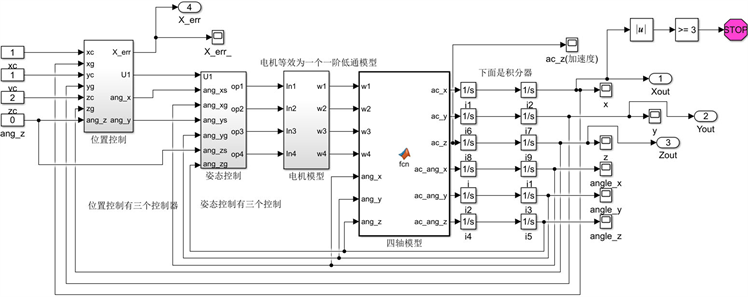5.2. 仿真结果比较分析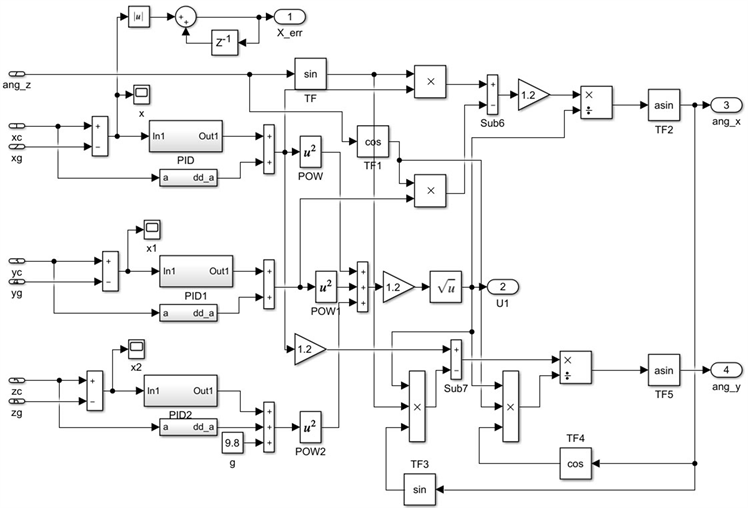Figure 9. Position control module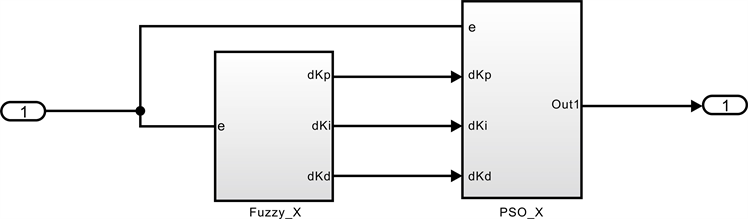Figure 10. Fuzzy adaptive PID controller based on PSO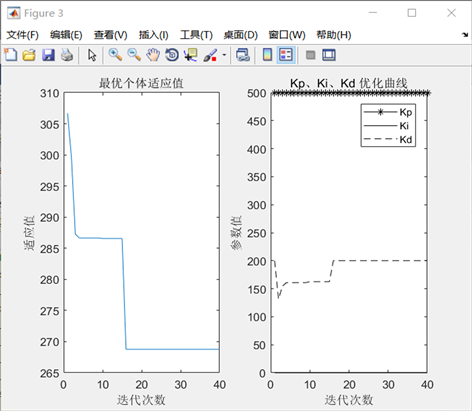Figure 11. Fitness curve with iteration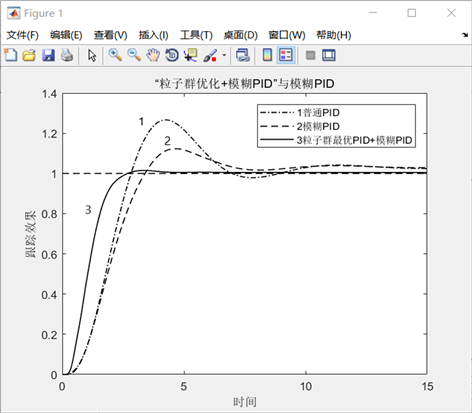Figure 12. Comparisons of simulation results

6. 结论

1) 普通PID控制在系统参数变化或外部扰动情况下，达不到理想的控制效果。

2) 模糊自适应PID控制，是一种可以在线整定的控制策略，可以根据偏差和偏差变化率的不同，调整PID参数，达到良好的控制效果。将模糊自适应PID控制应用到四旋翼悬停控制中，仿真结果验证了该控制方法的可行性，但是存在实际输出存在一定的稳态误差的问题。

3) 采用粒子群优化的模糊自适应PID控制器的策略，对系统PID参数进行优化，确保了四旋翼悬停控制系统的动态性能和稳定性，通过仿真验证了该控制方法可以使系统输出达到稳定、准确、快速的要求。相较于传统的控制方法，粒子群优化模糊自适应PID控制的方法具有稳定性好、调整速度快、鲁棒性强的特点。

 刘丽丽. 四旋翼飞行仿真器的建模及控制方法的研究[D]: [硕士学位论文]. 长沙: 中南大学, 2009.

 赵维斌. 四旋翼无人机飞行控制策略研究与优化[D]: [硕士学位论文]. 太原: 山西大学, 2017.

 聂博文, 马宏绪, 王剑, 王建文. 微小型四旋翼飞行器的研究现状与关键技术[J]. 电光与控制, 2007, 14(6): 113-117.

 胡彬杨. 无人机飞行控制方法研究与设计[D]: [硕士学位论文]. 北京: 电子科技大学, 2018.

 李俊, 李运堂. 四旋翼飞行器的动力学建模及PID控制[J]. 辽宁工程技术大学学报(自然科学版), 2012, 31(1): 114-117.

 吴成富, 刘小齐, 袁旭. 四旋翼无人机建模及其PID控制律设计[J]. 电子设计工程, 2012, 20(16): 68-70.

 郭佳晖, 任梦洁. 基于串级模糊PID的四旋翼姿态控制研究[J]. 电子设计工程, 2018, 26(17): 145-149.

 Limnaios, G. and Tsourveloudis, N. (2012) Fuzzy Logic Controller for a Mini Coaxial Indoor Helicopter. Journal of Intelligent & Robotic Systems, 65, 187-201.

 刘金琨. 先进PID控制MATLAB仿真[M]. 北京: 电子工业出版社, 2014.

 赵玉颖. 基于粒子群参数优化的四旋翼飞行器控制器设计研究[D]: [硕士学位论文]. 兰州: 兰州交通大学, 2017.

 Kennedy, J. (2011) Particle Swarm Optimization. In: Encyclopedia of Machine Learning, Springer, New York, 177-191.

 胡改玲, 桂亮, 权双璐, 郭婷, 王永泉, 王军平. 串联模糊PID控制的四旋翼无人机控制系统设计[J]. 实验技术与管理, 2019, 26(3): 132-135.

 李建国, 孟瑞锋, 董明飞, 候龚, 康立鹏. 四旋翼飞行器PID控制器的设计及仿真[J]. 南方农机, 2019, 50(7): 20-21.

Top